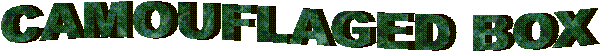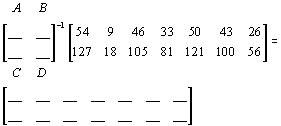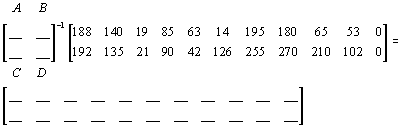Mathman 22 - The Clover Box by Jacob Eickstedt, Jonah Gauger, and Isaiah HoffTerrain: Grassy park and ATV trails Difficulty: Depends on math ability Placed by: The Dragon (Mathman) and his Honors Advanced Algebra students of 2005-06 Location: Jackson Town Hall in Jackson, WI County: Washington, WI Materials needed: Calculator, Compass, Writing Utensil, Personal Stamp, Inking PensStudents and parents of students should read my introduction to letterboxing before seeking the boxes.

These clues rely on the use of matrices to encode/decode clues.  Go to this page to learn how to do this on a free graphics calculator simulator or to do it by hand.

Be sure to read through all the clues before looking for the boxes so you know what is expected of you.

Bring bug spray in the non-winter months!!!!

Clues:

1.      Park in the parking lot. Start between the oak tree through the roof and the door to the north of it. (This Bur Oak is one of the largest in Wisconsin.)
Count the number of stars on the American flag: c = _____
Next count the number of red stripes on the flag: b = _____
Finally, count the number of small triangles on the door: a = _____
Solve the following equation for (d). This is the bearing you are to take.

2b + c = d/a

Now find the prefix (not area code) in the telephone number on the door: n=_____
Then count the number of white posts on the front of the building: w= _____
Add the three digits of n together and multiply the result by w: _____
This is the number of paces to take on the (d) bearing.

2.      When you get to this point, you should be lined up with a cupola, flagpole, and a square light post.
Count the number of square light posts in the lot: k = ______
and the number of lights on all these light posts: m = ______
Take m and square it. This will be the number of paces to take south.
(You'll use k later.)

3.      Soon, you will end up at an object, (a very round and heavy object)
Make note of the date z = _____ and year y = ______ on the object.
From there, count the number of rows of seats on one set of bleachers: b=_____
Then use (k) from above and solve the following equation for (x):

logbx = k

Now walk x paces on a bearing of x.

4.      When you get to this next point, turn to the west and count the number of blue silos off in the distance: s = _____  Now solve the following for v:

v = ms2 + 8s + z

Walk v paces to the east along the tree line.

5.      On the next object on the north side is a gold serial number, followed by a black on silver serial number. The last 4 digits are a,b,c,d in the following matrix. Decode the matrix and then, walk to the next point and you should be ready to play ball!6.    Make note of the large 2 digit number below the serial number: j = ____

7.      When you get there (or nearby - parallel the spot if being used), FOIL out the following equation. The constant term in the quadratic expression is the number of paces to take east.

(x + j)(x + s)

8.      When you get to this point, solve the matrix below, using the year on the object in clue 3 of this  box as a,b,c,d to decode the message and to find where to go next.9.   Follow the decoded message to the next object. Just in front of it is a mowed trail. Solve the following problem for k (the constant of variation):

y varies directly as x,  and y = 220, and x = π

"K" will be approximately the number of paces to go east on the trail.

10.  When you get to this point on the trail, find the discriminant of the following equation: y=3x2+7x-10, if the equation has 2 different real roots take the trail to the left, if the equation has 1 double root take the trail back the way you came, if the equation has complex roots turn right.

11. Then, walk 72 paces down that trail, and STOP!! Solve the following for the k - the constant of variation:

y varies inversely as x and y = 80 when x = 4

K is the bearing to take and you should walk y paces.

12.  You will then reach an intersection of trails.

Solve the following equation for the paces to take on the correct trail (round to the nearest whole number):

4.75=log3x.

Then add up the solutions in the following quadratic equation to get the bearing:

x2 + 46x - 1407 = 0

Note: the actual trail to stay on will go straight, then meander to the right, then meander to the left.  You will stop at a split in the path.

The point where you end with your paces STOP!! Solve the logarithm for the bearing (x) to take:

6=logb64

The abscissa is the number of paces on that trail. Then take 5 paces towards that many trunks to an ash tree.  On the north side of the tree, you will find the box hidden under a few rocks and bark.  When you are done hide the box well again, especially so it doesn't fall down the hill!

Before you set out read the waiver of responsibility and disclaimer.

LbNA Home